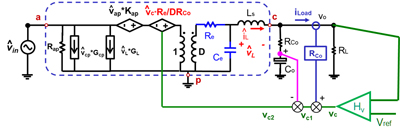Contact Us
LIBRARY

# Small Signal Analysis of V2 Control Using Current Mode Equivalent Circuit ModelFig. 1. Small signal equivalent Circuit of V2 Control.
In V2 control, the direct feedback contains the information of both state variables. In this paper, by separating current feedback and capacitor voltage feedback, an equivalent circuit of V2 control based on the equivalent circuit of current mode control is proposed. The proposed equivalent circuit provides a clear physical insight of V2 control. V2 control can be interpreted as an implementation of current mode control with direct voltage feedback and load current feedback. The load current feed-forward dramatically reduces the output impedance. The model is extended to enhanced V2 control. The proposed equivalent circuit model is applicable to both variable frequency modulation and constant frequency modulation.

The direct output voltage vo feedback consists of inductor current, capacitor voltage and load current feedback. Inductor current feedback loop does not have a low pass filter, so all the sideband frequencies (fsw-fm, fsw fm, etc.) are fed back to the modulator. Other feedbacks are simpler: for a voltage regulator, the impedance of the capacitor branch is much smaller than that of the load resistor at f>fsw/10. Assuming the capacitor ESR zero is well below half switching frequency, the impedance of ESR is much larger than that of the intrinsic capacitance, so the sideband components at vcap are overwhelmed by the ESR voltage.

According to the analysis above, it is reasonable to consider all the sideband frequency feedback effect in the inductor current loop, but only consider fundamental frequency in the capacitor voltage and load current feedback. The inductor current loop is a highly nonlinear entity. It potentially has sub-harmonic instability. A three-terminal switch equivalent circuit model based on the result that the describing function derivation accurately predicts the small signal properties of this nonlinear entity. Substitute the closed current loop and the PWM switch by the equivalent circuit mode, the proposed equivalent circuit model of V2 control is shown in Fig.1.
INDUSTRY PARTNERS